# 基于STM32F103ZET6的二维超声波风速测量系统设计Design of Two-Dimensional Ultrasonic Wind Speed Measurement System Based on STM32F103ZET6

DOI: 10.12677/JEE.2018.64033, PDF, HTML, XML, 下载: 535  浏览: 1,080

Abstract: The accuracy of wind speed measurement is important for the observation of the ocean-atmosphere interface. This paper briefly describes the advantages of the time difference ultrasonic wind speed measurement method, and based on the two-dimensional V-structure ultrasonic measurement principle of time difference method, a two-dimensional ultrasonic wind speed measurement system based on STM32F103ZET6 is built. Compared with traditional wind measurement systems, the design introduces the Hilbert Huang Transform algorithm to process the measurement data, which can ensure the accuracy of the wind speed measurement. The experimental results show that the design has strong adaptability and can achieve high precision wind speed measurement. It has high reference value and practical significance in the observation of complex meteorological environment such as air-sea interface.

1. 引言

2. 时间差测风方法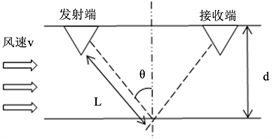Figure 1. V-type measurement schematic

$\frac{2L}{{t}_{1}}=c+v\mathrm{sin}\theta$ (1)

$\frac{2L}{{t}_{2}}=c-v\mathrm{sin}\theta$ (2)

$v=\frac{L}{\mathrm{sin}\theta }\left(\frac{1}{{t}_{1}}-\frac{1}{{t}_{2}}\right)$ (3)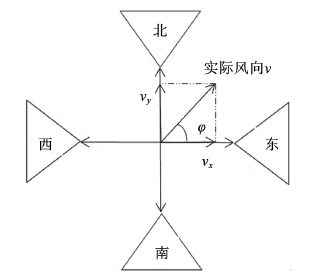Figure 2. Wind speed vector

${v}_{x}=\frac{L}{\mathrm{sin}\theta }\left(\frac{1}{{t}_{12}}-\frac{1}{{t}_{21}}\right)$ (4)

${v}_{y}=\frac{L}{\mathrm{sin}\theta }\left(\frac{1}{{t}_{34}}-\frac{1}{{t}_{43}}\right)$ (5)

$v=\frac{L}{\mathrm{sin}\theta }\sqrt{{\left(\frac{1}{{t}_{34}}-\frac{1}{{t}_{43}}\right)}^{2}+{\left(\frac{1}{{t}_{12}}-\frac{1}{{t}_{21}}\right)}^{2}}$ (6)

3. 系统方案设计

3.1. 整体方案设计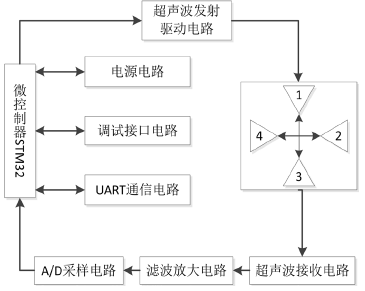Figure 3. System overall composition

3.2. 硬件设计

3.2.1. 超声波发射驱动模块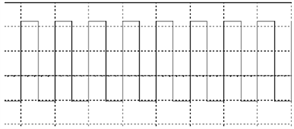Figure 4. Typical PWM waveform of the actual circuit

3.2.2. 超声波接收模块

LC选频电路如图5所示：左侧是一个74HC238D译码器，而右侧是发射、接收电路。1、2和3分别是由STM32F103ZET6控制的地址输入；K1、K2、K3和K4作为输出端。S1、S3与收、发兼具的声波探头相连接，CH1和CH3为收到的信号  。E1、E2、E3是译码器的势能点，连接控制器I/O口PB3与PB4。由S3侧的探头接收，测得CH3的回波信号数据并保存  。

3.2.3. 信号采集保存

3.3. HHT算法简介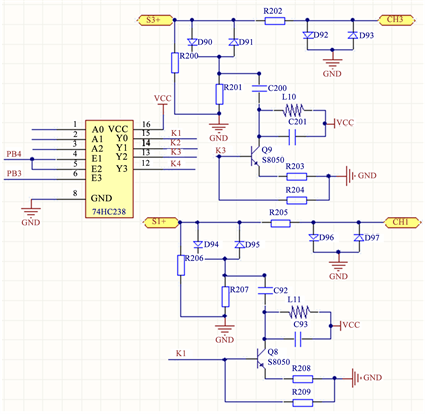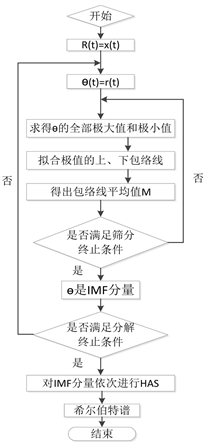Figure 6. HHT flow chart

1) 基于原始信号 $x\left(t\right)$ ，通过三次样条插值法处理极大值和极小值点，得到上、下包络线的平均值为：

${r}_{1}\left(t\right)=\frac{1}{2}\left({\alpha }_{1}\left(t\right)+{\alpha }_{2}\left(t\right)\right)$ (7)

2) 计算原始信号 $x\left(t\right)$ 与平均值 ${r}_{1}\left(t\right)$ 的差值：

${\theta }_{1}\left(t\right)=x\left(t\right)-{r}_{1}\left(t\right)$ (8)

3) 如果满足IMF条件，那么分解停止；如果不满足IMF条件，那么将上述步骤重复直到满足条件，令剩余分量为 ${r}_{1}\left(t\right)$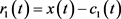(9)

4) 用剩余分量作为新的信号反复以上的步骤，分离出来所有的IMF分量与剩余分量的关系如下：

$\left\{\begin{array}{l}{r}_{1}-{c}_{2}={r}_{2}\\ M\\ {r}_{n-1}-{c}_{n}={r}_{n}\end{array}$ (10)

4. 实验结果与分析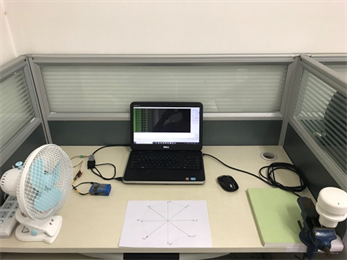Figure 7. System test physical chart

4.1. 实验结论

1) 在低风速情况下，采用时差法超声波测风原理能够分别测量出超声波换能器接收到的顺风和逆风时的超声波回波信号，从而测量出风速值；

2) 基于一定数量的试验，运用HHT算法进行数据处理，验证了本文设计的具有较高精确度和稳定性。已知空气中的波速340 m/s，本设计里两对换能器之间传输声程均是140 mm，可得传播时间间隔为0.41 ms  ；相比较而言，STM32F的最高时钟为72 MHz，可知时间精确度最高可达14 ns。Figure 8. Downwind wave receiving signal waveform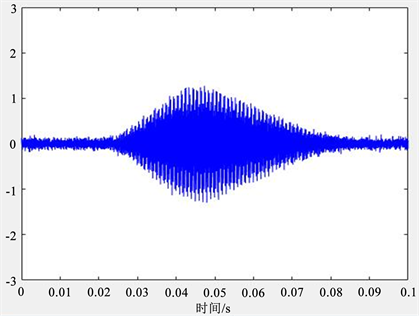Figure 9. Upwind direction ultrasonic receiving signal waveform

4.2. 误差的原因分析

1) 受电路板面积的影响，实时的温度补偿电路并没有添加，而是将测得的外部环境温度写入程序中，从而将测量误差引入系统  ；

2) 用时间差法测量出的低风速值精度基本满足要求。但是在中、高风速情况下，还需要进一步的验证测试  。

5. 结束语

1) 外界温湿度也会影响风速，可以添加温湿度补偿器来增强测风仪的性能；

2) 在数据处理方面引入新的算法来提高准确性。

  陈志洲, 刘小河, 田雨聪. 基于 STM32 单片机测风系统的设计[J]. 电气技术, 2016(5): 31-35+44.  刘德荣, 王瑛, 王铁流, 张炳洋. 基于STM32F的超声波风速风向仪设计[J]. 测控技术, 2017, 36(6): 97-100.  温玉波．海洋环境基础数据格式转换的研究[D]: [硕士学位论文]. 青岛: 中国海洋大学, 2014．  李聪, 国红玉, 王峰. 二维超声波风速仪[J]. 仪表技术与传感, 2015(2): 29-32.  梁家怡, 超声波二维风速风向测量系统设计[D]: [硕士学位论文]. 哈尔滨: 哈尔滨理工大学, 2013．  崔国恒, 赵金超, 曹可劲, 等. 基于凹面反射的超声风速测量系统设计与实现[J]. 声学技术, 2014, 33(4): 336-340.  行鸿彦, 于祥, 邹水平, 等. 风杯式风速传感器启动风速校准实验箱的分析与设计[J]. 仪器仪表学报, 2015, 36(9): 1996-2004.  高中华, 赵湛, 杜利东, 等. 超声波风速风向仪的电路设计[J]. 仪表技术与传感器, 2014(1): 19-20.  杨帆. 嵌入式系统在智能便携式自动气象站中的研究与应用[D]: [硕士学位论文]. 南京: 南京航空航天大学, 2012.  全鹏程, 易仕和, 武宇, 等. 激波与层流/湍流边界层相互作用实验研究[J]. 物理学报, 2014, 63(8): 239-243.  Kim, M. (2015) Test Bench Development for Acquisition Module FPGA of Ultrasonic Flow Meter. 2015 International Conference on Pervasive Computing, 1-6.  Bucci, G., Ciancetta, F., Fiorucci, E., et al. (2013) A Low-Cost Ultrasonic Wind Speed and Direction Measurement System. 2013 IEEE International Instrumentation and Measurement Technology Conference, 505-510.  陈子建, 王振涛, 许杰斌. EMD 技术在船舶液压设备故障诊断中的应用研究[J]. 机械工程与技术, 2017, 6(4): 324-335.